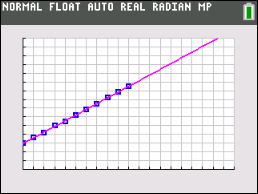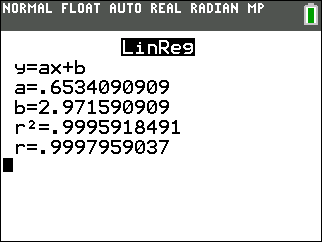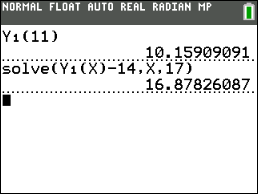# Activities

••• ##### Subject Area

• Math: Middle Grades Math: Statistics and Probability

• ##### Author6-8

45 Minutes

• ##### Device
• TI-83 Plus Family
• TI-84 Plus
• TI-84 Plus Silver Edition
•TI-84 Plus C Silver Edition
•TI-84 Plus CE

## The Marble Experiment#### Activity Overview

Students determine, by examining a graph, if a data set has a positive or negative correlation coefficient.

#### Key Steps

•Students will graph a scatterplot of data and determine a linear function that describes the data.

•Students will determine the equation of a line of best fit to analyze and make predictions about data.Students will graph a scatterplot of data and determine a linear function that describes the data.

•Students will interpret the real-world significance of the slope and y-intercept of a linear model.